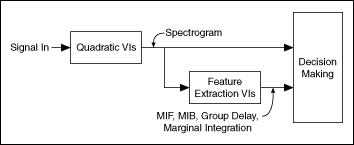LabVIEW 2014 Advanced Signal Processing Toolkit Help

Edition Date: June 2014

Part Number: 372656C-01

»View Product InfoDownload Help (Windows Only)

In traditional spectral analysis, the discrete Fourier transform and the inverse discrete Fourier transform are complementary operations. The discrete Fourier transform computes a frequency-domain representation of time-domain signals. The inverse discrete Fourier transform converts the frequency-domain representation back to the time-domain representation.

One important application of the Fourier transform is computing the power spectrum of a signal. The power spectrum presents the energy of a signal as a function of frequency. You can use the power spectrum to detect harmonics of a signal and to examine the frequency response of a system when the spectral content does not change over time. Because the power spectrum usually is estimated by the square of the Fourier transform, the power spectrum is considered a quadratic frequency analysis method.

For signals with spectral content that changes over time, the quadratic time-frequency analysis methods compute the energy of a signal as a function of time and frequency, resulting in a quadratic time-frequency representation of the signal. Because a quadratic time-frequency representation approximately describes the energy density of a signal in the time-frequency domain, the time-frequency representation is called a distribution or a spectrogram.Note  This help refers to distributions and spectrograms as spectrograms.

Use the TFA Configure Spectrogram Indicator VI to display a spectrogram on an intensity graph as a color map, from which you can determine the spectral content of a signal and how the spectral content evolves over time. You also can save the time-dependent 2D array to a text file for use in another software environment. The resulting text file contains only Z values and does not retain the time axis information or the frequency axis information. Use the TFA Get Time and Freq Scale Info VI to compute the time scale information and the frequency scale information of the time-frequency representation.

From a spectrogram, you also can extract features from a signal, such as the mean instantaneous frequency (MIF) and the group delay. You can use the information in these extracted features in signal analysis, detection, estimation, and classification.

Unlike the linear time-frequency analysis methods, the quadratic time-frequency analysis methods are not invertible, meaning you cannot reconstruct time-domain signals from spectrograms. If you need to reconstruct signals, use the linear time-frequency analysis methods instead.

The quadratic time-frequency analysis method you select depends on the requirements of the application. Consider the resolution, the negative values, the cross-term interference, and computation speed when you select a quadratic time-frequency analysis method.

The following table compares these properties of the quadratic time-frequency analysis methods in the Time Frequency Analysis Tools. In general, most applications use the STFT spectrogram and the Gabor spectrogram.

Method Resolution Includes Negative Values? Includes Cross-Term Interference? Speed
Short-time Fourier transform (STFT) spectrogram, including the STFT-based reassignment method Coarse No No Fast
Wigner-Ville distribution (WVD) Fine Yes Yes, strong Fast
Choi-Williams distribution (CWD) Moderate Yes Suppresses cross-terms that two auto-terms with different time centers and frequency centers generate but does not suppress cross-terms that two auto-terms with the same time center or frequency center generate Very slow
Cone-shaped distribution (CSD) Moderate Yes Suppresses cross-terms that two auto-terms with different time centers and frequency centers generate but does not reduce cross-terms that two auto-terms with the same time center generate Very slow
Gabor spectrogram Fine Yes Minor when order is small Moderate
Adaptive spectrogram Best for signal of chirplets No No Depends on signal length, number of components to extract

The following figure shows the typical steps for creating applications for signal analysis, detection, and classification using the quadratic time-frequency analysis method VIs.You can use the Time Frequency Analysis VIs to compute the spectrogram of a signal, analyze the spectrogram directly, and then decide what step to take next. You also can use the spectrogram to compute other indexes, such as the MIF, the mean instantaneous bandwidth (MIB), the group delay, and the marginal integration before deciding what step to take next.